# Ch9-Gauss_Elimination4.pdf

29 Mar 2022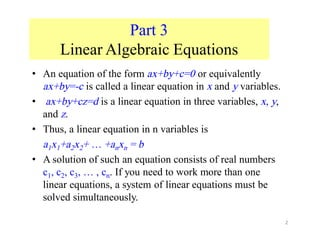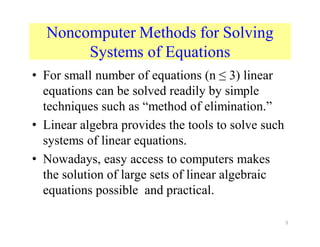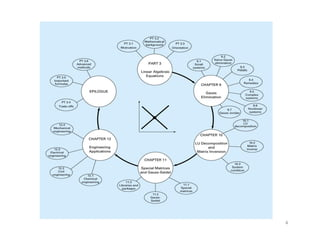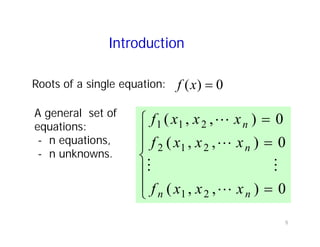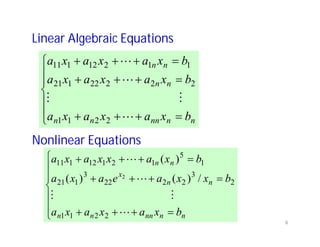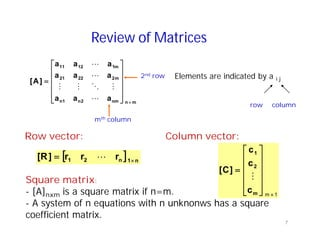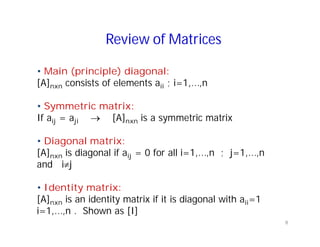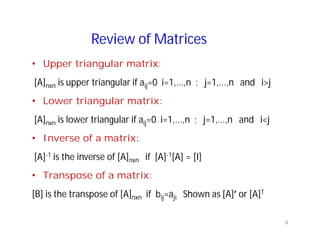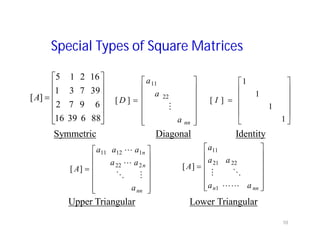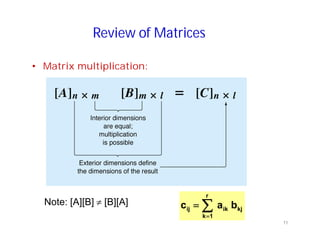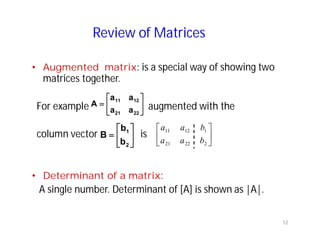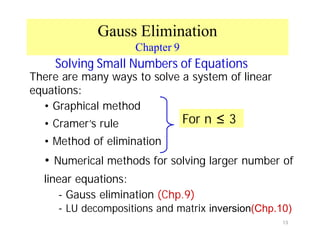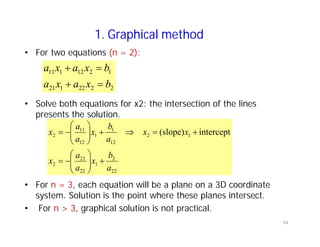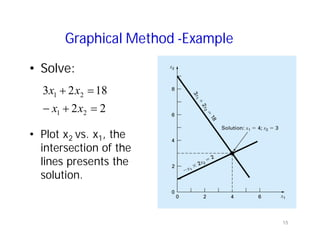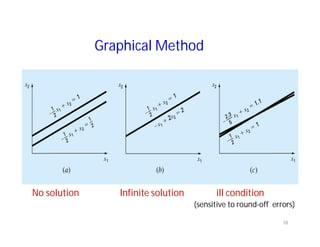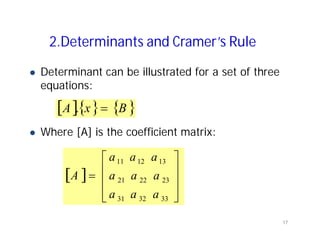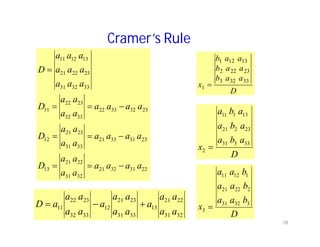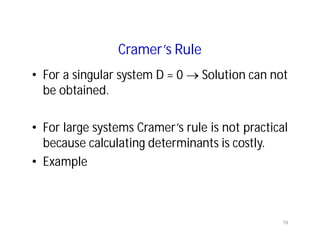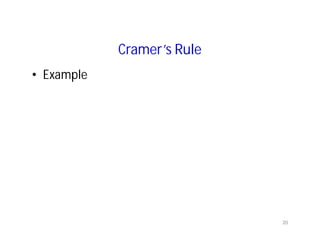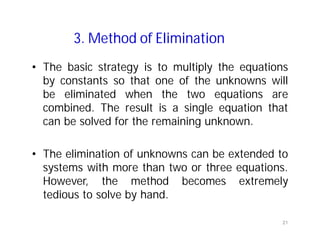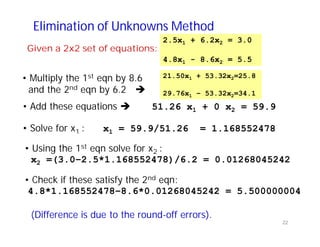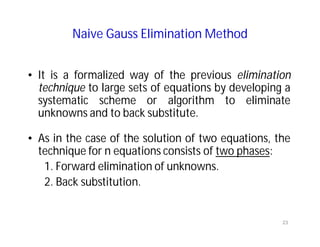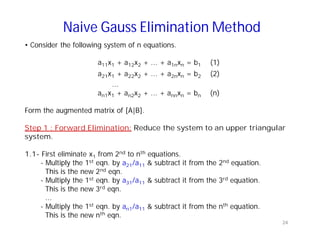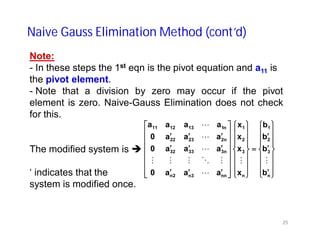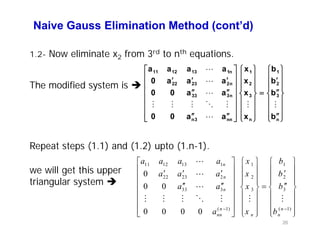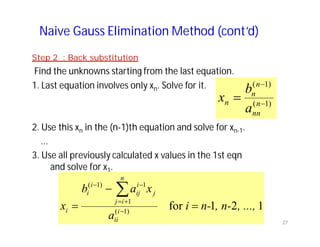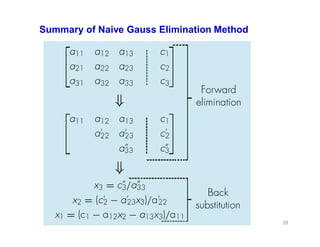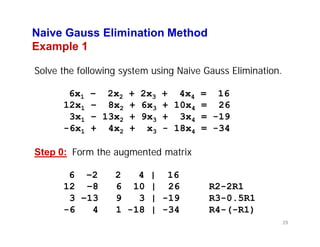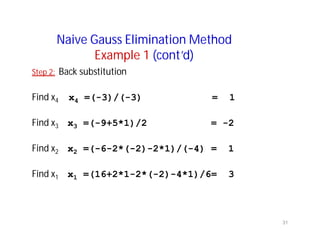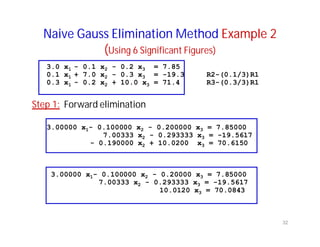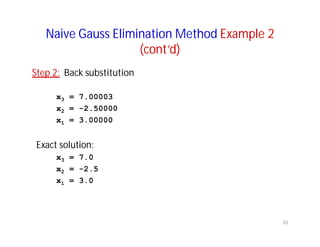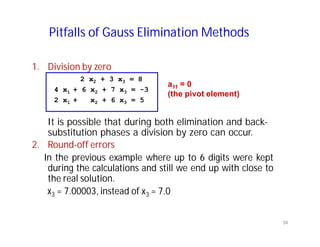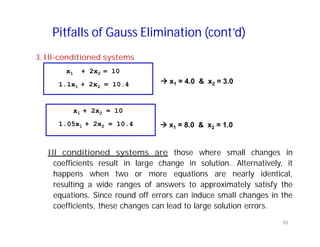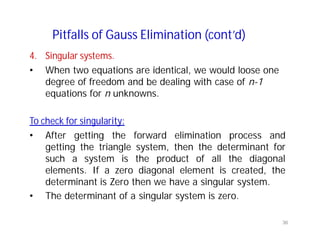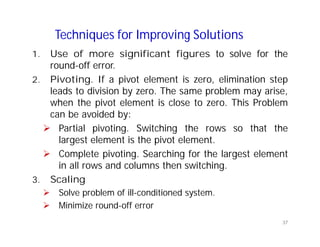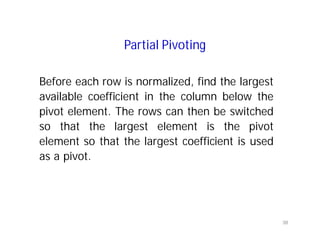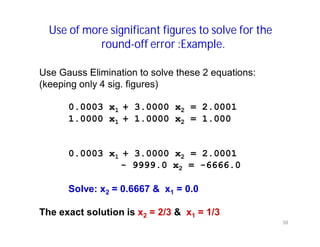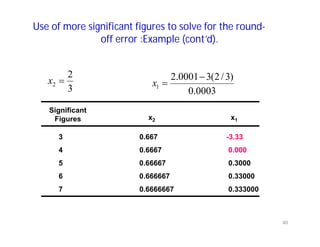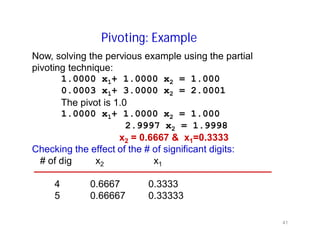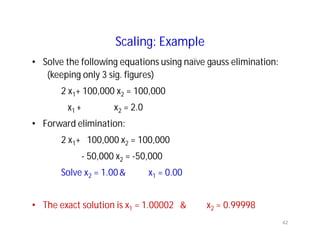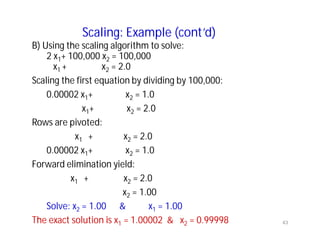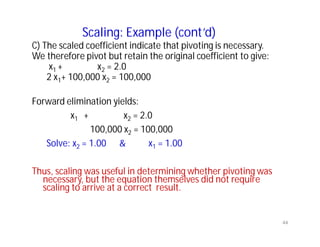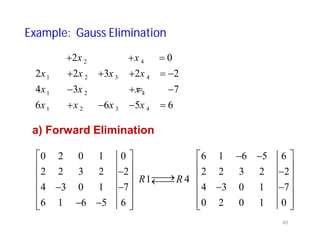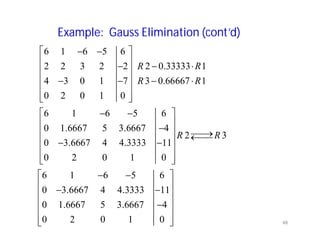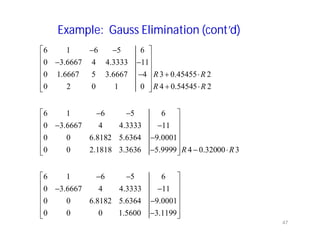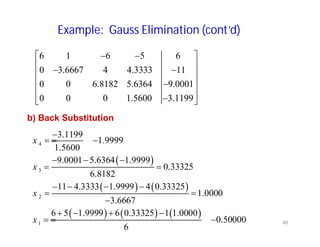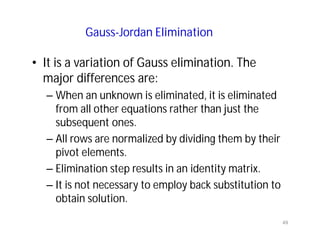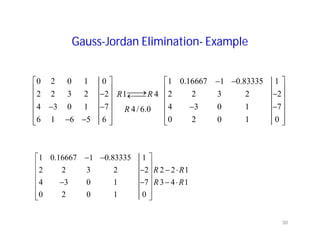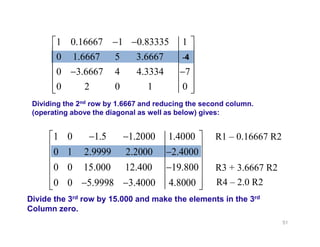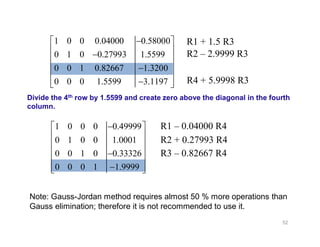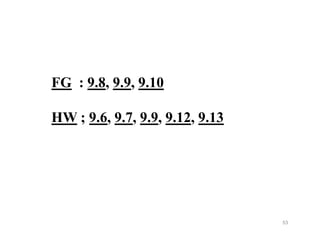1 sur 53

### Ch9-Gauss_Elimination4.pdf

• 1. The Islamic University of Gaza Faculty of Engineering Civil Engineering Department Numerical Analysis ECIV 3306 Chapter 9 Gauss Elimination 1
• 2. Part 3 Linear Algebraic Equations • An equation of the form ax+by+c=0 or equivalently ax+by=-c is called a linear equation in x and y variables. • ax+by+cz=d is a linear equation in three variables, x, y, and z. • Thus, a linear equation in n variables is a1x1+a2x2+ … +anxn = b • A solution of such an equation consists of real numbers c1, c2, c3, … , cn. If you need to work more than one linear equations, a system of linear equations must be solved simultaneously. 2
• 3. Noncomputer Methods for Solving Systems of Equations • For small number of equations (n 3) linear equations can be solved readily by simple techniques such as “method of elimination.” • Linear algebra provides the tools to solve such systems of linear equations. • Nowadays, easy access to computers makes the solution of large sets of linear algebraic equations possible and practical. 3
• 4. 4
• 5. Introduction 0 ) (x f Roots of a single equation: A general set of equations: - n equations, - n unknowns. 0 ) , , ( 0 ) , , ( 0 ) , , ( 2 1 2 1 2 2 1 1 n n n n x x x f x x x f x x x f 5
• 6. Linear Algebraic Equations n n nn n n n n x n n b x a x a x a b x x a e a x a b x a x x a x a 2 2 1 1 2 3 2 2 22 3 1 21 1 5 1 2 1 12 1 11 / ) ( ) ( ) ( 2 n n nn n n n n n n b x a x a x a b x a x a x a b x a x a x a 2 2 1 1 2 2 2 22 1 21 1 1 2 12 1 11 Nonlinear Equations 6
• 7. Review of Matrices m n nm 2 n 1 n m 2 22 21 m 1 12 11 a a a a a a a a a ] A [ 2nd row mth column Elements are indicated by a i j row column Row vector: Column vector: n 1 n 2 1 r r r ] R [ 1 m m 2 1 c c c ] C [ Square matrix: - [A]nxm is a square matrix if n=m. - A system of n equations with n unknonws has a square coefficient matrix. 7
• 8. Review of Matrices • Main (principle) diagonal: [A]nxn consists of elements aii ; i=1,...,n • Symmetric matrix: If aij = aji [A]nxn is a symmetric matrix • Diagonal matrix: [A]nxn is diagonal if aij = 0 for all i=1,...,n ; j=1,...,n and i j • Identity matrix: [A]nxn is an identity matrix if it is diagonal with aii=1 i=1,...,n . Shown as [I] 8
• 9. Review of Matrices • Upper triangular matrix: [A]nxn is upper triangular if aij=0 i=1,...,n ; j=1,...,n and i>j • Lower triangular matrix: [A]nxn is lower triangular if aij=0 i=1,...,n ; j=1,...,n and i<j • Inverse of a matrix: [A]-1 is the inverse of [A]nxn if [A]-1[A] = [I] • Transpose of a matrix: [B] is the transpose of [A]nxn if bij=aji Shown as [A] or [A]T 9
• 10. Special Types of Square Matrices 88 6 39 16 6 9 7 2 39 7 3 1 16 2 1 5 ] [A nn a a a D 22 11 ] [ 1 1 1 1 ] [ I Symmetric Diagonal Identity nn n n a a a a a a A] [ 2 22 1 12 11 nn n a a a a a A 1 22 21 11 ] [ Upper Triangular Lower Triangular 10
• 11. Review of Matrices • Matrix multiplication: r 1 k kj ik ij b a c Note: [A][B] [B][A] 11
• 12. Review of Matrices • Augmented matrix: is a special way of showing two matrices together. For example augmented with the column vector is • Determinant of a matrix: A single number. Determinant of [A] is shown as |A|. 22 21 12 11 a a a a A 2 1 b b B 2 22 21 1 12 11 b a a b a a 12
• 13. Solving Small Numbers of Equations There are many ways to solve a system of linear equations: • Graphical method • Cramer’s rule • Method of elimination • Numerical methods for solving larger number of linear equations: - Gauss elimination (Chp.9) - LU decompositions and matrix inversion(Chp.10) For n 3 13 Gauss Elimination Chapter 9
• 14. 1. Graphical method • For two equations (n = 2): • Solve both equations for x2: the intersection of the lines presents the solution. • For n = 3, each equation will be a plane on a 3D coordinate system. Solution is the point where these planes intersect. • For n > 3, graphical solution is not practical. 2 2 22 1 21 1 2 12 1 11 b x a x a b x a x a 22 2 1 22 21 2 1 2 12 1 1 12 11 2 intercept (slope) a b x a a x x x a b x a a x 14
• 15. Graphical Method -Example • Solve: • Plot x2 vs. x1, the intersection of the lines presents the solution. 2 2 18 2 3 2 1 2 1 x x x x 15
• 16. Graphical Method No solution Infinite solution ill condition (sensitive to round-off errors) 16
• 17. 2.Determinants and Cramer’s Rule Determinant can be illustrated for a set of three equations: Where [A] is the coefficient matrix: B x A . 33 32 31 23 22 21 13 12 11 a a a a a a a a a A 17
• 18. Cramer’s Rule 22 31 32 21 32 31 22 21 13 23 31 33 21 33 31 23 21 12 23 32 33 22 33 32 23 22 11 33 32 31 23 22 21 13 12 11 a a a a a a a a D a a a a a a a a D a a a a a a a a D a a a a a a a a a D 32 31 22 21 13 33 31 23 21 12 33 32 23 22 11 a a a a a a a a a a a a a a a D D a a b a a b a a b x 33 32 3 23 22 2 13 12 1 1 D a b a a b a a b a x 33 3 31 23 2 21 13 1 1 1 2 D b a a b a a b a a x 3 32 31 2 22 21 1 12 11 3 18
• 19. Cramer’s Rule • For a singular system D = 0 Solution can not be obtained. • For large systems Cramer’s rule is not practical because calculating determinants is costly. • Example 19
• 20. Cramer’s Rule • Example 20
• 21. 3. Method of Elimination • The basic strategy is to multiply the equations by constants so that one of the unknowns will be eliminated when the two equations are combined. The result is a single equation that can be solved for the remaining unknown. • The elimination of unknowns can be extended to systems with more than two or three equations. However, the method becomes extremely tedious to solve by hand. 21
• 22. Elimination of Unknowns Method Given a 2x2 set of equations: 2.5x1 + 6.2x2 = 3.0 4.8x1 - 8.6x2 = 5.5 • Multiply the 1st eqn by 8.6 and the 2nd eqn by 6.2 21.50x1 + 53.32x2=25.8 29.76x1 – 53.32x2=34.1 • Add these equations 51.26 x1 + 0 x2 = 59.9 • Solve for x1 : x1 = 59.9/51.26 = 1.168552478 • Using the 1st eqn solve for x2 : x2 =(3.0–2.5*1.168552478)/6.2 = 0.01268045242 • Check if these satisfy the 2nd eqn: 4.8*1.168552478–8.6*0.01268045242 = 5.500000004 (Difference is due to the round-off errors). 22
• 23. Naive Gauss Elimination Method • It is a formalized way of the previous elimination technique to large sets of equations by developing a systematic scheme or algorithm to eliminate unknowns and to back substitute. • As in the case of the solution of two equations, the technique for n equations consists of two phases: 1. Forward elimination of unknowns. 2. Back substitution. 23
• 24. Naive Gauss Elimination Method • Consider the following system of n equations. a11x1 + a12x2 + ... + a1nxn = b1 (1) a21x1 + a22x2 + ... + a2nxn = b2 (2) ... an1x1 + an2x2 + ... + annxn = bn (n) Form the augmented matrix of [A|B]. Step 1 : Forward Elimination: Reduce the system to an upper triangular system. 1.1- First eliminate x1 from 2nd to nth equations. - Multiply the 1st eqn. by a21/a11 & subtract it from the 2nd equation. This is the new 2nd eqn. - Multiply the 1st eqn. by a31/a11 & subtract it from the 3rd equation. This is the new 3rd eqn. ... - Multiply the 1st eqn. by an1/a11 & subtract it from the nth equation. This is the new nth eqn. 24
• 25. Note: - In these steps the 1st eqn is the pivot equation and a11 is the pivot element. - Note that a division by zero may occur if the pivot element is zero. Naive-Gauss Elimination does not check for this. The modified system is ‘ indicates that the system is modified once. n 3 2 1 n 3 2 1 nn 3 n 2 n n 3 33 32 n 2 23 22 n 1 13 12 11 b b b b x x x x a a a 0 a a a 0 a a a 0 a a a a Naive Gauss Elimination Method (cont’d) 25
• 26. Naive Gauss Elimination Method (cont’d) 1.2- Now eliminate x2 from 3rd to nth equations. The modified system is n 3 2 1 n 3 2 1 nn 3 n n 3 33 n 2 23 22 n 1 13 12 11 b b b b x x x x a a 0 0 a a 0 0 a a a 0 a a a a Repeat steps (1.1) and (1.2) upto (1.n-1). 11 12 13 1 1 1 22 23 2 2 2 33 3 3 3 ( 1) ( 1) 0 0 0 0 0 0 0 n n n n n nn n n a a a a x b a a a x b a a x b a x b we will get this upper triangular system 26
• 27. Naive Gauss Elimination Method (cont’d) Step 2 : Back substitution Find the unknowns starting from the last equation. 1. Last equation involves only xn. Solve for it. 2. Use this xn in the (n-1)th equation and solve for xn-1. ... 3. Use all previously calculated x values in the 1st eqn and solve for x1. ) 1 ( ) 1 ( n nn n n n a b x 1 2 1 for ) 1 ( 1 1 ) 1 ( , ..., , n- n- i a x a b x i ii n i j j i ij i i i 27
• 28. Summary of Naive Gauss Elimination Method 28
• 29. Naive Gauss Elimination Method Example 1 Solve the following system using Naive Gauss Elimination. 6x1 – 2x2 + 2x3 + 4x4 = 16 12x1 – 8x2 + 6x3 + 10x4 = 26 3x1 – 13x2 + 9x3 + 3x4 = -19 -6x1 + 4x2 + x3 - 18x4 = -34 Step 0: Form the augmented matrix 6 –2 2 4 | 16 12 –8 6 10 | 26 R2-2R1 3 –13 9 3 | -19 R3-0.5R1 -6 4 1 -18 | -34 R4-(-R1) 29
• 30. Naive Gauss Elimination Method Example 1 (cont’d) Step 1: Forward elimination 1. Eliminate x1 6 –2 2 4 | 16 (Does not change. Pivot is 6) 0 –4 2 2 | -6 0 –12 8 1 | -27 R3-3R2 0 2 3 -14 | -18 R4-(-0.5R2) 2. Eliminate x2 6 –2 2 4 | 16 (Does not change.) 0 –4 2 2 | -6 (Does not change. Pivot is-4) 0 0 2 -5 | -9 0 0 4 -13 | -21 R4-2R3 3. Eliminate x3 6 –2 2 4 | 16 (Does not change.) 0 –4 2 2 | -6 (Does not change.) 0 0 2 -5 | -9 (Does not change. Pivot is 2) 0 0 0 -3 | -3 30
• 31. Naive Gauss Elimination Method Example 1 (cont’d) Step 2: Back substitution Find x4 x4 =(-3)/(-3) = 1 Find x3 x3 =(-9+5*1)/2 = -2 Find x2 x2 =(-6-2*(-2)-2*1)/(-4) = 1 Find x1 x1 =(16+2*1-2*(-2)-4*1)/6= 3 31
• 32. Naive Gauss Elimination Method Example 2 (Using 6 Significant Figures) 3.0 x1 - 0.1 x2 - 0.2 x3 = 7.85 0.1 x1 + 7.0 x2 - 0.3 x3 = -19.3 R2-(0.1/3)R1 0.3 x1 - 0.2 x2 + 10.0 x3 = 71.4 R3-(0.3/3)R1 Step 1: Forward elimination 3.00000 x1- 0.100000 x2 - 0.200000 x3 = 7.85000 7.00333 x2 - 0.293333 x3 = -19.5617 - 0.190000 x2 + 10.0200 x3 = 70.6150 3.00000 x1- 0.100000 x2 - 0.20000 x3 = 7.85000 7.00333 x2 - 0.293333 x3 = -19.5617 10.0120 x3 = 70.0843 32
• 33. Naive Gauss Elimination Method Example 2 (cont’d) Step 2: Back substitution x3 = 7.00003 x2 = -2.50000 x1 = 3.00000 Exact solution: x3 = 7.0 x2 = -2.5 x1 = 3.0 33
• 34. Pitfalls of Gauss Elimination Methods 1. Division by zero 2 x2 + 3 x3 = 8 4 x1 + 6 x2 + 7 x3 = -3 2 x1 + x2 + 6 x3 = 5 It is possible that during both elimination and back- substitution phases a division by zero can occur. 2. Round-off errors In the previous example where up to 6 digits were kept during the calculations and still we end up with close to the real solution. x3 = 7.00003, instead of x3 = 7.0 a11 = 0 (the pivot element) 34
• 35. Pitfalls of Gauss Elimination (cont’d) 3. Ill-conditioned systems x1 + 2x2 = 10 1.1x1 + 2x2 = 10.4 x1 + 2x2 = 10 1.05x1 + 2x2 = 10.4 Ill conditioned systems are those where small changes in coefficients result in large change in solution. Alternatively, it happens when two or more equations are nearly identical, resulting a wide ranges of answers to approximately satisfy the equations. Since round off errors can induce small changes in the coefficients, these changes can lead to large solution errors. x1 = 4.0 & x2 = 3.0 x1 = 8.0 & x2 = 1.0 35
• 36. Pitfalls of Gauss Elimination (cont’d) 4. Singular systems. • When two equations are identical, we would loose one degree of freedom and be dealing with case of n-1 equations for n unknowns. To check for singularity: • After getting the forward elimination process and getting the triangle system, then the determinant for such a system is the product of all the diagonal elements. If a zero diagonal element is created, the determinant is Zero then we have a singular system. • The determinant of a singular system is zero. 36
• 37. Techniques for Improving Solutions 1. Use of more significant figures to solve for the round-off error. 2. Pivoting. If a pivot element is zero, elimination step leads to division by zero. The same problem may arise, when the pivot element is close to zero. This Problem can be avoided by: Partial pivoting. Switching the rows so that the largest element is the pivot element. Complete pivoting. Searching for the largest element in all rows and columns then switching. 3. Scaling Solve problem of ill-conditioned system. Minimize round-off error 37
• 38. Partial Pivoting Before each row is normalized, find the largest available coefficient in the column below the pivot element. The rows can then be switched so that the largest element is the pivot element so that the largest coefficient is used as a pivot. 38
• 39. Use of more significant figures to solve for the round-off error :Example. Use Gauss Elimination to solve these 2 equations: (keeping only 4 sig. figures) 0.0003 x1 + 3.0000 x2 = 2.0001 1.0000 x1 + 1.0000 x2 = 1.000 0.0003 x1 + 3.0000 x2 = 2.0001 - 9999.0 x2 = -6666.0 Solve: x2 = 0.6667 & x1 = 0.0 The exact solution is x2 = 2/3 & x1 = 1/3 39
• 40. Use of more significant figures to solve for the round- off error :Example (cont’d). 3 2 2 x 0003 . 0 ) 3 / 2 ( 3 0001 . 2 1 x Significant Figures x2 x1 3 0.667 -3.33 4 0.6667 0.000 5 0.66667 0.3000 6 0.666667 0.33000 7 0.6666667 0.333000 40
• 41. Pivoting: Example Now, solving the pervious example using the partial pivoting technique: 1.0000 x1+ 1.0000 x2 = 1.000 0.0003 x1+ 3.0000 x2 = 2.0001 The pivot is 1.0 1.0000 x1+ 1.0000 x2 = 1.000 2.9997 x2 = 1.9998 x2 = 0.6667 & x1=0.3333 Checking the effect of the # of significant digits: # of dig x2 x1 4 0.6667 0.3333 5 0.66667 0.33333 41
• 42. Scaling: Example • Solve the following equations using naïve gauss elimination: (keeping only 3 sig. figures) 2 x1+ 100,000 x2 = 100,000 x1 + x2 = 2.0 • Forward elimination: 2 x1+ 100,000 x2 = 100,000 - 50,000 x2 = -50,000 Solve x2 = 1.00& x1 = 0.00 • The exact solution is x1 = 1.00002 & x2 = 0.99998 42
• 43. Scaling: Example (cont’d) B) Using the scaling algorithm to solve: 2 x1+ 100,000 x2 = 100,000 x1 + x2 = 2.0 Scaling the first equation by dividing by 100,000: 0.00002 x1+ x2 = 1.0 x1+ x2 = 2.0 Rows are pivoted: x1 + x2 = 2.0 0.00002 x1+ x2 = 1.0 Forward elimination yield: x1 + x2 = 2.0 x2 = 1.00 Solve: x2 = 1.00 & x1 = 1.00 The exact solution is x1 = 1.00002 & x2 = 0.99998 43
• 44. Scaling: Example (cont’d) C) The scaled coefficient indicate that pivoting is necessary. We therefore pivot but retain the original coefficient to give: x1 + x2 = 2.0 2 x1+ 100,000 x2 = 100,000 Forward elimination yields: x1 + x2 = 2.0 100,000 x2 = 100,000 Solve: x2 = 1.00 & x1 = 1.00 Thus, scaling was useful in determining whether pivoting was necessary, but the equation themselves did not require scaling to arrive at a correct result. 44
• 45. Example: Gauss Elimination 2 4 1 2 3 4 1 2 4 1 2 3 4 2 0 2 2 3 2 2 4 3 7 6 6 5 6 x x x x x x x x x x x x x a) Forward Elimination 0 2 0 1 0 6 1 6 5 6 2 2 3 2 2 2 2 3 2 2 1 4 4 3 0 1 7 4 3 0 1 7 6 1 6 5 6 0 2 0 1 0 R R 45
• 46. Example: Gauss Elimination (cont’d) 6 1 6 5 6 2 2 3 2 2 2 0.33333 1 4 3 0 1 7 3 0.66667 1 0 2 0 1 0 6 1 6 5 6 0 1.6667 5 3.6667 4 2 3 0 3.6667 4 4.3333 11 0 2 0 1 0 6 1 6 5 6 0 3.6667 4 4.3333 11 0 1.6667 5 3.6667 4 0 2 0 1 0 R R R R R R 46
• 47. Example: Gauss Elimination (cont’d) 6 1 6 5 6 0 3.6667 4 4.3333 11 0 1.6667 5 3.6667 4 3 0.45455 2 0 2 0 1 0 4 0.54545 2 6 1 6 5 6 0 3.6667 4 4.3333 11 0 0 6.8182 5.6364 9.0001 0 0 2.1818 3.3636 5.9999 4 0.32000 3 6 1 6 0 3.6667 4 0 0 6.8 0 0 R R R R R R 5 6 4.3333 11 182 5.6364 9.0001 0 1.5600 3.1199 47
• 48. Example: Gauss Elimination (cont’d) b) Back Substitution 6 1 6 5 6 0 3.6667 4 4.3333 11 0 0 6.8182 5.6364 9.0001 0 0 0 1.5600 3.1199 4 3 2 1 3.1199 1.9999 1.5600 9.0001 5.6364 1.9999 0.33325 6.8182 11 4.3333 1.9999 4 0.33325 1.0000 3.6667 6 5 1.9999 6 0.33325 1 1.0000 0.50000 6 x x x x 48
• 49. Gauss-Jordan Elimination • It is a variation of Gauss elimination. The major differences are: – When an unknown is eliminated, it is eliminated from all other equations rather than just the subsequent ones. – All rows are normalized by dividing them by their pivot elements. – Elimination step results in an identity matrix. – It is not necessary to employ back substitution to obtain solution. 49
• 50. Gauss-Jordan Elimination- Example 0 2 0 1 0 1 0.16667 1 0.83335 1 2 2 3 2 2 2 2 3 2 2 1 4 4 3 0 1 7 4 3 0 1 7 4/ 6.0 6 1 6 5 6 0 2 0 1 0 R R R 1 0.16667 1 0.83335 1 2 2 3 2 2 2 2 1 4 3 0 1 7 3 4 1 0 2 0 1 0 R R R R 50
• 51. 1 0.16667 1 0.83335 1 0 1.6667 5 3.6667 2 0 3.6667 4 4.3334 7 0 2 0 1 0 Dividing the 2nd row by 1.6667 and reducing the second column. (operating above the diagonal as well as below) gives: 1 0 1.5 1.2000 1.4000 0 1 2.9999 2.2000 2.4000 0 0 15.000 12.400 19.800 0 0 5.9998 3.4000 4.8000 Divide the 3rd row by 15.000 and make the elements in the 3rd Column zero. 51 R1 – 0.16667 R2 R3 + 3.6667 R2 R4 – 2.0 R2 -4
• 52. 1 0 0 0.04000 0.58000 0 1 0 0.27993 1.5599 0 0 1 0.82667 1.3200 0 0 0 1.5599 3.1197 Divide the 4th row by 1.5599 and create zero above the diagonal in the fourth column. 1 0 0 0 0.49999 0 1 0 0 1.0001 0 0 1 0 0.33326 0 0 0 1 1.9999 Note: Gauss-Jordan method requires almost 50 % more operations than Gauss elimination; therefore it is not recommended to use it. 52 R1 + 1.5 R3 R2 – 2.9999 R3 R4 + 5.9998 R3 R1 – 0.04000 R4 R2 + 0.27993 R4 R3 – 0.82667 R4
• 53. FG : 9.8, 9.9, 9.10 HW ; 9.6, 9.7, 9.9, 9.12, 9.13 53